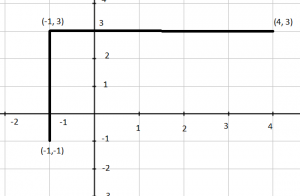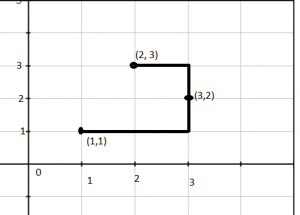# Number of horizontal or vertical line segments to connect 3 points

Given three points on the x-y coordinate plane. You need to find the no. of line segments formed by making a polyline passing through these points. (Line segment can be vertically or horizontally aligned to the coordinate axis)

Examples :

```Input : A  = {-1, -1}, B = {-1, 3}, C = {4, 3}
Output :   2
Expantaion:There are two segments in this polyline.
Input :A = {1, 1}, B = {2, 3} C = {3, 2}
Output : 3```

## Recommended: Please try your approach on {IDE} first, before moving on to the solution.

The result is one if all points are on x axis or y axis. The result is 2 if points can form L shape. L shape is formed if any of the three points can be used as a joining point. Otherwise answer is 3.

## C++

 `// CPP program to find number of horizontal (or vertical ` `// line segments needed to connect three points. ` `#include ` `using` `namespace` `std; ` ` `  `// Function to check if the third point forms a  ` `// rectangle with other two points at corners ` `bool` `isBetween(``int` `a, ``int` `b, ``int` `c)  ` `{ ` `    ``return` `min(a, b) <= c && c <= max(a, b); ` `} ` ` `  `// Returns true if point k can be used as a joining ` `// point to connect using two line segments ` `bool` `canJoin(``int` `x[], ``int` `y[], ``int` `i, ``int` `j, ``int` `k)  ` `{ ` `    ``// Check for the valid polyline with two segments ` `    ``return` `(x[k] == x[i] || x[k] == x[j]) &&  ` `                ``isBetween(y[i], y[j], y[k]) || ` `        ``(y[k] == y[i] || y[k] == y[j]) &&  ` `                ``isBetween(x[i], x[j], x[k]); ` `} ` ` `  `int` `countLineSegments(``int` `x[], ``int` `y[]) ` `{ ` `    ``// Check whether the X-coordinates or  ` `    ``// Y-cocordinates are same.  ` `    ``if` `((x == x && x == x) || ` `        ``(y == y && y == y)) ` `        ``return` `1; ` ` `  `    ``// Iterate over all pairs to check for two ` `    ``// line segments ` `    ``else` `if` `(canJoin(x, y, 0, 1, 2) || ` `            ``canJoin(x, y, 0, 2, 1) ||  ` `            ``canJoin(x, y, 1, 2, 0)) ` `        ``return` `2; ` ` `  `    ``// Otherwise answer is three. ` `    ``else` `        ``return` `3; ` `} ` ` `  `// Driver code ` `int` `main() ` `{ ` `    ``int` `x, y; ` `    ``x = -1; y = -1; ` `    ``x = -1; y = 3; ` `    ``x = 4; y = 3; ` `    ``cout << countLineSegments(x, y); ` `    ``return` `0; ` `} `

## Java

 `// Java program to find number of horizontal ` `// (or vertical line segments needed to ` `// connect three points. ` `import` `java.io.*; ` ` `  `class` `GFG { ` `     `  `// Function to check if the third ` `// point forms a rectangle with  ` `// other two points at corners ` `static` `boolean` `isBetween(``int` `a, ``int` `b, ``int` `c)  ` `{ ` `    ``return` `(Math.min(a, b) <= c && ` `                    ``c <= Math.max(a, b)); ` `} ` ` `  `// Returns true if point k can be  ` `// used as a joining point to connect ` `// using two line segments ` `static` `boolean` `canJoin(``int` `x[], ``int` `y[], ` `                        ``int` `i, ``int` `j, ``int` `k)  ` `{ ` `    ``// Check for the valid polyline  ` `    ``// with two segments ` `    ``return` `(x[k] == x[i] || x[k] == x[j]) &&  ` `                ``isBetween(y[i], y[j], y[k]) || ` `                ``(y[k] == y[i] || y[k] == y[j]) &&  ` `                ``isBetween(x[i], x[j], x[k]); ` `} ` ` `  `static` `int` `countLineSegments(``int` `x[], ``int` `y[]) ` `{ ` `    ``// Check whether the X-coordinates or  ` `    ``// Y-cocordinates are same.  ` `    ``if` `((x[``0``] == x[``1``] && x[``1``] == x[``2``]) || ` `        ``(y[``0``] == y[``1``] && y[``1``] == y[``2``])) ` `        ``return` `1``; ` ` `  `    ``// Iterate over all pairs to check for two ` `    ``// line segments ` `    ``else` `if` `(canJoin(x, y, ``0``, ``1``, ``2``) || ` `            ``canJoin(x, y, ``0``, ``2``, ``1``) ||  ` `            ``canJoin(x, y, ``1``, ``2``, ``0``)) ` `        ``return` `2``; ` ` `  `    ``// Otherwise answer is three. ` `    ``else` `        ``return` `3``; ` `} ` ` `  `// Driver code ` `public` `static` `void` `main (String[] args) { ` ` `  `    ``int` `x[]=``new` `int``[``3``], y[]=``new` `int``[``3``]; ` `     `  `    ``x[``0``] = -``1``; y[``0``] = -``1``; ` `    ``x[``1``] = -``1``; y[``1``] = ``3``; ` `    ``x[``2``] = ``4``; y[``2``] = ``3``; ` `     `  `    ``System.out.println(countLineSegments(x, y)); ` `    ``} ` `     `  `     `  `} ` ` `  `// This code is contributed by vt_m `

## Python3

 `# Python program to find number ` `# of horizontal (or vertical ` `# line segments needed to ` `# connect three points. ` ` `  `import` `math ` ` `  `# Function to check if the ` `# third point forms a  ` `# rectangle with other ` `# two points at corners ` `def` `isBetween(a, b, c) : ` ` `  `    ``return` `min``(a, b) <``=` `c ``and` `c <``=` `max``(a, b) ` ` `  `  `  `# Returns true if point k ` `# can be used as a joining ` `# point to connect using ` `# two line segments ` `def` `canJoin( x, y, i, j, k) : ` ` `  `    ``# Check for the valid polyline ` `    ``# with two segments ` `    ``return` `(x[k] ``=``=` `x[i] ``or` `x[k] ``=``=` `x[j]) ``and` `isBetween(y[i], y[j], y[k]) ``or` `(y[k] ``=``=` `y[i] ``or` `y[k] ``=``=` `y[j]) ``and` `isBetween(x[i], x[j], x[k]) ` ` `  `  `  `def` `countLineSegments( x, y): ` ` `  `    ``# Check whether the X-coordinates or  ` `    ``# Y-cocordinates are same.  ` `    ``if` `((x[``0``] ``=``=` `x[``1``] ``and` `x[``1``] ``=``=` `x[``2``]) ``or` `        ``(y[``0``] ``=``=` `y[``1``] ``and` `y[``1``] ``=``=` `y[``2``])): ` `        ``return` `1` `  `  `    ``# Iterate over all pairs to check for two ` `    ``# line segments ` `    ``elif` `(canJoin(x, y, ``0``, ``1``, ``2``) ``or` `            ``canJoin(x, y, ``0``, ``2``, ``1``) ``or`  `            ``canJoin(x, y, ``1``, ``2``, ``0``)): ` `        ``return` `2` `  `  `    ``# Otherwise answer is three. ` `    ``else``: ` `        ``return` `3` `#driver code ` `x``=` `[``-``1``,``-``1``, ``4``] ` `y``=` `[``-``1``, ``3``, ``3``] ` ` `  `print``(countLineSegments(x, y)) ` ` `  `# This code is contributed by Gitanjali. `

## C#

 `// C# program to find number of horizontal ` `// (or vertical) line segments needed to ` `// connect three points. ` `using` `System; ` ` `  `class` `GFG { ` ` `  `    ``// Function to check if the third ` `    ``// point forms a rectangle with ` `    ``// other two points at corners ` `    ``static` `bool` `isBetween(``int` `a, ``int` `b, ``int` `c) ` `    ``{ ` `        ``return` `(Math.Min(a, b) <= c &&  ` `                          ``c <= Math.Max(a, b)); ` `    ``} ` ` `  `    ``// Returns true if point k can be ` `    ``// used as a joining point to connect ` `    ``// using two line segments ` `    ``static` `bool` `canJoin(``int``[] x, ``int``[] y, ` `                        ``int` `i, ``int` `j, ``int` `k) ` `    ``{ ` `         `  `        ``// Check for the valid polyline ` `        ``// with two segments ` `        ``return` `(x[k] == x[i] || x[k] == x[j])  ` `               ``&& isBetween(y[i], y[j], y[k])  ` `               ``|| (y[k] == y[i] || y[k] == y[j])  ` `               ``&& isBetween(x[i], x[j], x[k]); ` `    ``} ` ` `  `    ``static` `int` `countLineSegments(``int``[] x, ``int``[] y) ` `    ``{ ` `         `  `        ``// Check whether the X-coordinates or ` `        ``// Y-cocordinates are same. ` `        ``if` `((x == x && x == x) || ` `                  ``(y == y && y == y)) ` `            ``return` `1; ` ` `  `        ``// Iterate over all pairs to check for two ` `        ``// line segments ` `        ``else` `if` `(canJoin(x, y, 0, 1, 2)  ` `                      ``|| canJoin(x, y, 0, 2, 1)  ` `                      ``|| canJoin(x, y, 1, 2, 0)) ` `            ``return` `2; ` ` `  `        ``// Otherwise answer is three. ` `        ``else` `            ``return` `3; ` `    ``} ` ` `  `    ``// Driver code ` `    ``public` `static` `void` `Main() ` `    ``{ ` ` `  `        ``int``[] x = ``new` `int``; ` `        ``int``[] y = ``new` `int``; ` ` `  `        ``x = -1; ` `        ``y = -1; ` `        ``x = -1; ` `        ``y = 3; ` `        ``x = 4; ` `        ``y = 3; ` ` `  `        ``Console.WriteLine(countLineSegments(x, y)); ` `    ``} ` `} ` ` `  `// This code is contributed by vt_m. `

## PHP

 ` `

Output :

```  2
```My Personal Notes arrow_drop_upCheck out this Author's contributed articles.

If you like GeeksforGeeks and would like to contribute, you can also write an article using contribute.geeksforgeeks.org or mail your article to contribute@geeksforgeeks.org. See your article appearing on the GeeksforGeeks main page and help other Geeks.

Please Improve this article if you find anything incorrect by clicking on the "Improve Article" button below.

Improved By : vt_m